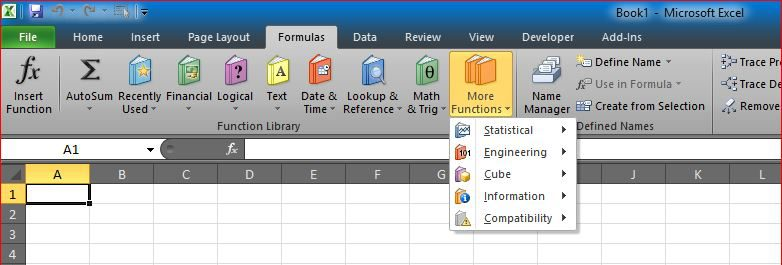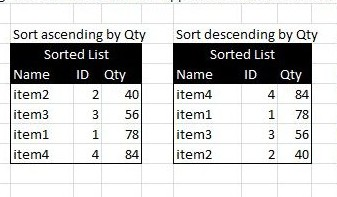# Sort list dynamically (functions)Sorting a list automatically using formulas, with no need to press the sort command
Also if the source table is changed, the destination table will do also.

What you need is basically two formulas, one for the sort-by column to list items by order. Use SMALL to sort ascending, or LARGE to sort descending, then another formula to retrieve other columns data based on the sort-by column.

Sort-by column (Ascending order):

`=SMALL(\$D\$8:\$D\$11,ROW()-7)`

Sort-by column (Descending order):

`=LARGE(\$D\$8:\$D\$11,ROW()-7)`

And you can either use a regular VLOOKUP to get other columns data (assuming the sort-by column is the first column in  original list, or, you can use INDEX with MATCH to get all columns not mater where is the sort-by column located, like this one:

`=INDEX(\$B\$8:\$D\$11,MATCH(L8,\$D\$8:\$D\$11,0),1)`

Screenshots and attached file should be clear to see and modify…